Open in App
Not now

# Python | Introduction to Matplotlib

• Difficulty Level : Medium
• Last Updated : 22 Mar, 2023

Matplotlib is an amazing visualization library in Python for 2D plots of arrays. Matplotlib is a multi-platform data visualization library built on NumPy arrays and designed to work with the broader SciPy stack. It was introduced by John Hunter in the year 2002. One of the greatest benefits of visualization is that it allows us visual access to huge amounts of data in easily digestible visuals. Matplotlib consists of several plots like line, bar, scatter, histogram etc. Installation : Windows, Linux and macOS distributions have matplotlib and most of its dependencies as wheel packages. Run the following command to install matplotlib package :

`python -mpip install -U matplotlib`

Importing matplotlib :

` `

#### Basic plots in Matplotlib :

Matplotlib comes with a wide variety of plots. Plots helps to understand trends, patterns, and to make correlations. They’re typically instruments for reasoning about quantitative information. Some of the sample plots are covered here. Line plot :

## Python3

 `# importing matplotlib module ` `from` `matplotlib ``import` `pyplot as plt`   `# x-axis values` `x ``=` `[``5``, ``2``, ``9``, ``4``, ``7``]`   `# Y-axis values` `y ``=` `[``10``, ``5``, ``8``, ``4``, ``2``]`   `# Function to plot` `plt.plot(x,y)`   `# function to show the plot` `plt.show()`

Output :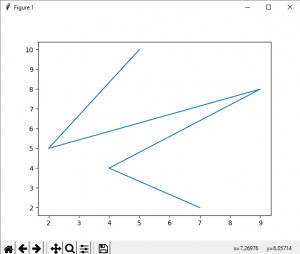Bar plot :

## Python3

 `# importing matplotlib module ` `from` `matplotlib ``import` `pyplot as plt`   `# x-axis values` `x ``=` `[``5``, ``2``, ``9``, ``4``, ``7``]`   `# Y-axis values` `y ``=` `[``10``, ``5``, ``8``, ``4``, ``2``]`   `# Function to plot the bar` `plt.bar(x,y)`   `# function to show the plot` `plt.show()`

Output :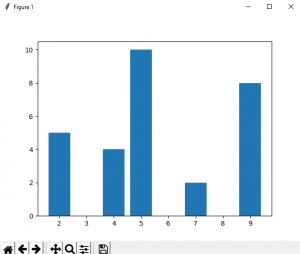Histogram :

## Python3

 `# importing matplotlib module ` `from` `matplotlib ``import` `pyplot as plt`   `# Y-axis values` `y ``=` `[``10``, ``5``, ``8``, ``4``, ``2``]`   `# Function to plot histogram` `plt.hist(y)`   `# Function to show the plot` `plt.show()`

Output :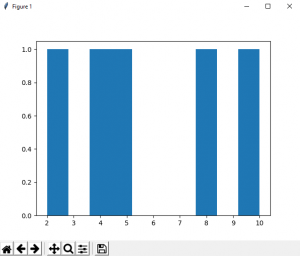Scatter Plot :

## Python3

 `# importing matplotlib module ` `from` `matplotlib ``import` `pyplot as plt`   `# x-axis values` `x ``=` `[``5``, ``2``, ``9``, ``4``, ``7``]`   `# Y-axis values` `y ``=` `[``10``, ``5``, ``8``, ``4``, ``2``]`   `# Function to plot scatter` `plt.scatter(x, y)`   `# function to show the plot` `plt.show()`

Output :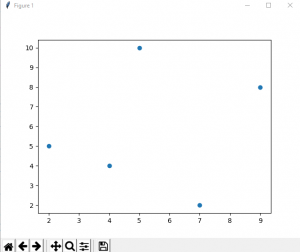We can also plot a scatter plot using the plot() method by providing the marker style as ‘o’.

## Python3

 `# importing matplotlib module ` `from` `matplotlib ``import` `pyplot as plt`   `# x-axis values` `x ``=` `[``5``, ``2``, ``9``, ``4``, ``7``]`   `# Y-axis values` `y ``=` `[``10``, ``5``, ``8``, ``4``, ``2``]`   `# Function to plot scatter using plot() method` `plt.plot(x,y,``'o'``)`       `# function to show the plot` `plt.show()`

Output –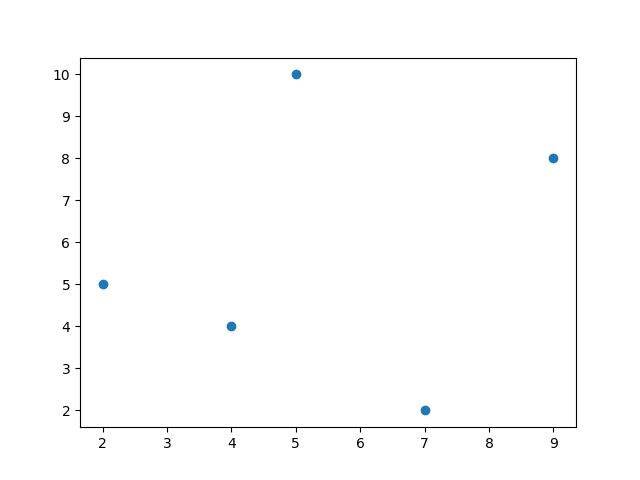Reference : Matplotlib Documentation.

My Personal Notes arrow_drop_up
Related Articles